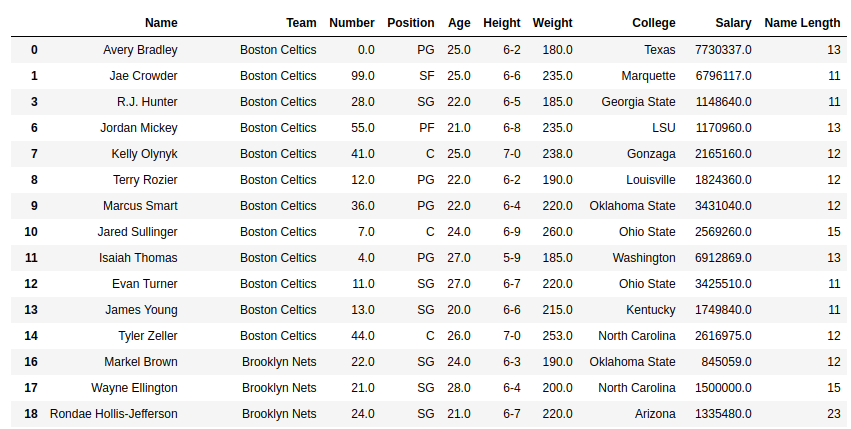# Python | Pandas Series.str.len()

Python is a great language for doing data analysis, primarily because of the fantastic ecosystem of data-centric Python packages. Pandas is one of those packages and makes importing and analyzing data much easier.

Pandas `str.len()` method is used to determine length of each string in a Pandas series. This method is only for series of strings.
Since this is a string method, .str has to be prefixed everytime before calling this method. Otherwise it will give an error.

Syntax: Series.str.len()

Return type: Series of integer values. NULL values might be present too depending upon caller series.

In the following examples, the data frame used contains data of some NBA players. The image of data frame before any operations is attached below.Example #1: Calculating length of string series (dtype=str)

In this example, the string length of Name column is calculated using str.len() method. The dtype of the Series is already string. So there is no need of data type conversion. Before doing any operations, null rows are removed to avoid errors.

 `# importing pandas module  ` `import` `pandas as pd ` ` `  `# reading csv file from url  ` `data ``=` `pd.read_csv(``"https://media.geeksforgeeks.org/wp-content/uploads/nba.csv"``) ` `  `  `# dropping null value columns to avoid errors ` `data.dropna(inplace ``=` `True``) ` ` `  `# creating new column for len ` `# passing values through str.len() ` `data[``"Name Length"``]``=` `data[``"Name"``].``str``.``len``() ` ` `  `# display ` `data `

Output:
As shown in the output image, the length of each string in name column is returned.Note:

• This method doesn’t count length of integer or float series. It will give an error since it’s not a string series. The series need to be converted first ( Shown in next Example)
• There is no parameter to handle null values. A null value would return null in the output string too.

Example #2:
In this example, the length of salary column is calculated using the str.len() method. Since the series is imported as float64 dtype, it’s first converted to string using .astype() method.

 `# importing pandas module  ` `import` `pandas as pd ` ` `  `# reading csv file from url  ` `data ``=` `pd.read_csv(``"https://media.geeksforgeeks.org/wp-content/uploads/nba.csv"``) ` `  `  `# dropping null value columns to avoid errors ` `data.dropna(inplace ``=` `True``) ` ` `  `# converting to string dtype ` `data[``"Salary"``]``=` `data[``"Salary"``].astype(``str``) ` ` `  `# passing values ` `data[``"Salary Length"``]``=` `data[``"Salary"``].``str``.``len``() ` ` `  `# converting back to float dtype ` `data[``"Salary"``]``=` `data[``"Salary"``].astype(``float``) ` ` `  `# display ` `data `

Output:
As shown in the output, length of int or float series can only be computed by converting it to string dtype.